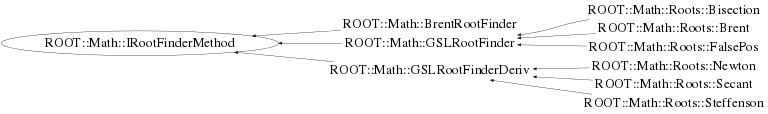# class ROOT::Math::IRootFinderMethod

```
Interface for finding function roots of one-dimensional functions

@ingroup RootFinders

```

## Function Members (Methods)

This is an abstract class, constructors will not be documented.
Look at the header to check for available constructors.

public:
 virtual ~IRootFinderMethod() ROOT::Math::IRootFinderMethod IRootFinderMethod() ROOT::Math::IRootFinderMethod IRootFinderMethod(const ROOT::Math::IRootFinderMethod&) virtual int Iterate() virtual int Iterations() const virtual const char* Name() const ROOT::Math::IRootFinderMethod& operator=(const ROOT::Math::IRootFinderMethod&) virtual double Root() const virtual bool SetFunction(const ROOT::Math::IGradFunction&, double) virtual bool SetFunction(const ROOT::Math::IGenFunction&, double, double) virtual bool Solve(int maxIter = 100, double absTol = 1.0E-8, double relTol = 1.0E-10) virtual int Status() const

## Class Charts## Function documentation

virtual ~IRootFinderMethod()
``` Default Destructor.
```
`{}`

``` Default Constructor.
```
`{}`
bool SetFunction(const ROOT::Math::IGradFunction& , double )
``` Common functionality
Sets the function for algorithms using derivatives.
```
bool SetFunction(const ROOT::Math::IGenFunction& , double , double )
``` Sets the function for the rest of the algorithms.
The parameters set the interval where the root has to be calculated.
```
double Root() const
``` Returns the previously calculated root.
```
int Status() const
``` Returns the status of the previous estimate
```
bool Solve(int maxIter = 100, double absTol = 1.0E-8, double relTol = 1.0E-10)
``` Methods to be Implemented in the derived classes
Stimates the root for the function.
\@param maxIter maximum number of iterations.
\@param absTol desired absolute error in the minimum position.
\@param absTol desired relative error in the minimum position.

```
const char* Name() const
``` Return name of root finder algorithm
```
int Iterate()
``` This method is  implemented only by the GSLRootFinder
and GSLRootFinderDeriv classes and will return an error if it's not one of them.
```
int Iterations() const
``` Return number of iterations used to find the root
Must be implemented by derived classes

```
`{ return -1; }`